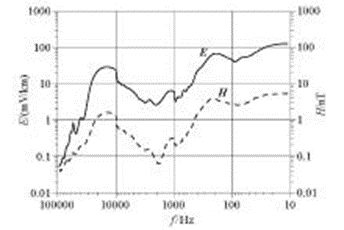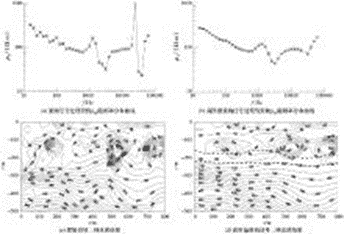# High frequency magnetotelluric noise suppression#(adsbygoogle = window.adsbygoogle || []).push({});

## Summary

The
high-frequency magnetotelluric (HMT) signal is easily disturbed by human noise
in the frequency range of 20 kHz to 100 kHz, the electromagnetic signal
strength is weak, and the data error is large. This paper proposes to use
high-order spectral analysis to reconstruct the high-frequency magnetotelluric
non-minimum phase signal, thereby effectively suppressing random Gaussian noise
and improving the signal-to-noise ratio. Through the processing of
numerical simulation signal and measured data, it is proved that the method can
effectively suppress the noise, and it is better than the traditional method in
extracting the actual geological information.

Key words : high-frequency
magnetotelluric method ; high-order spectrum ; signal
processing ; noise suppression

## Abstract

In
the frequency range of 20kHz ~ 100kHz, the HMT signal has the characteristics
of weak strength, low correlation and poor repeatability, which is caused by
the interference of human noise in the near surface. In this paper, the authors
used higher-order spectrum method to analyze and reconstruct HMT signal, which
can effectively suppress Gaussian random noise and raise signal noise ratio.
Numerical analog signal and measured data processing prove that the method is
feasible. According to the application effect, the method is trade better the
colored noise suppression and practical geological information extraction.

Keyword : high
frequency magnetotelluric method ; higher-order spectrum ; signal
processing ; noise suppression

## 0 Preface

The
high-frequency magnetotelluric method (HMT) was proposed according to the
engineering geological exploration needs of deep-buried tunnels. The
engineering survey is most concerned with the stratum geological
conditions within 1000 m near the surface[  ] . It is 10 Hz~100 kHz, which is
different from the traditional magnetotelluric (MT) observation frequency band
of 0.001~340 Hz and the audio frequency magnetotelluric method (AMT)
observation frequency band of 0.1 Hz~ n kHz [ ] . The electromagnetic method
is distinguished, which is defined as the high-frequency magnetotelluric
method. At present, the high-frequency magnetotelluric method can be
realized by using the electromagnetic observation system EH-4 conductivity
tensor measuring instrument jointly developed and produced by GEOMETRICS and
EMI. In recent years, the author has selected nearly a hundred
representative measuring points in several provinces and cities in southeastern
China, measured the electromagnetic fields in the 10 Hz-100 kHz frequency band,
and calculated the average amplitude strength of natural electromagnetic fields
in southeastern China (Fig. 1 ). The results show that In the
frequency range of 20 kHz to 100 kHz, the signal strength of the electric field
and magnetic field weakens as a whole, and the correlation of the electromagnetic
field signal decreases and the repeatability is poor. This is because
high-frequency signals are easily interfered by human noise, such as
high-voltage wires, electric railways, and telecommunications grounding
equipment. The complex and diverse noise effects often make the repeatability
of observations worse, and the estimation of impedance is scattered, which
cannot objectively reflect the underground electrical properties. distribution,
it may even lead to erroneous results.

Wang
Shuming et al.  believe that the magnetotelluric signal is non-Gaussian,
non-minimum phase and non-linear, that is, a “three non-” signal, and
the classical spectrum estimation method loses the phase information, and is
sensitive to high-frequency human noise suppression is not ideal. The
author tried to reconstruct the original time series by using the high-order
spectral estimation method to suppress the colored Gaussian noise, and then
calculate the resistivity and phase. From the perspective of application effect,
this method is better than the traditional method in suppressing the colored
noise and extracting the actual geological information.Figure 1 The average amplitude of high-frequency electromagnetic field strength in southeastern China

### 1 Analysis of high-order spectrum of HMT signal

Suppose
the signal x ( k ) is a k -th order
stationary random process with zero mean , then the k -th
order moment kx ( τ 1 ,
…, τ k- 1 ) of the process
is defined as

The
k-
order cumulant of the
process ( τ 1 , …, τ k- 1 )
is defined as

where: k ≥
3, g ( n ) is a Gaussian process with
the same power spectrum as x ( n ).

Thus, the
third-order cumulant of x ( k ) signal is

where E {
} represents mathematical expectation. After Fourier transform, formula
(1) becomes

(2)

Equation (2) is the third-order spectrum of x ( k )
, also called bispectrum, where ω the frequency. The
third-order spectrum has symmetry, namely:

From
formula (2), we can know that the phase spectrum of the bispectrum is

#### The amplitude spectrum is

where ϕ x ( ω )
and |X ( ω ) | are the phase
spectrum and amplitude spectrum of x ( k ) ,
respectively .

2 High-order spectrum estimation
to reconstruct the power spectrum

## 2.1 Amplitude estimation

By
taking the logarithm on both sides of formula (5), we can get

Substituting
equation (6) as a variable, that is, ω +ω =i , ω =j , ω =ij ,
the discrete expression can be obtained

When
taking i= 0, j= 0, we can get

### 2.2 Phase Estimation

There
are many methods for bispectrum phase estimation [ ] , and the BMU algorithm is
used in this paper. Brillinger first proposed a method to recursively
calculate ϕ ( ω ) from ϕ ( ω 1 , ω 2 )
, the starting point relation is

In
order to facilitate the operation and improve the calculation accuracy,
Matsuoka and Ulrych derived the discrete form of equation (10), in the
region

ω 1 + ω ω (0≤ω ≤ω ; 0≤ω ≤ω ) ,
further in the ω = [0, ω ]
summation,

let ω
-ω
1 get

For
the convenience of calculation, let Δ ω = 1, ω =i , ω =j , ω
=n
, we can get

(11)

in,

therefore,

Change
the above formula into recursive form, define

then
there is

where n=N corresponds
to ω = π. The initial value of the formula is

So
far, we have extracted the amplitude and phase from the third-order spectrum of
the magnetotelluric signal, obtained the signal spectrum, reconstructed the
signal through inverse Fourier transform, and then estimated the power spectrum
to obtain the impedance tensor components, apparent Resistivity and phase
information.

## 3 Theoretical and measured data processing and analysis

### 3.1 Numerical simulation analysis

The
effect of high-order spectral technique on suppressing random noise of HMT
signal is tested by numerical simulation synthetic data. Digital
simulation of high-frequency magnetotelluric high-frequency signals: the number
of sampling points is 4096, and the sampling frequency is 12 kHz, which
contains sinusoidal signals with frequencies of 30, 50, 100, 200, 300, 1000,
and 2000 Hz, using the matlab program rpiid function Gaussian noise, nonlinear
drift and DC components are added.

Figure
2a is the synthetic signal of numerical simulation, and Figure
2b ~d are the amplitude spectrum and phase spectrum of the Fourier
transform of the original signal and the reconstructed signal,
respectively. It can be seen from Fig. 2b that although the
original signal can distinguish 7 fundamental frequencies through Fourier
transform, its anti-interference performance is very poor, and the frequency spectrum
above 100 Hz is seriously disturbed by noise, among which 1 000 and 2 000 Hz
are basically submerged in the in the noise. It can be seen from Fig.
2d that the phase spectrum noise of the original signal is severely
interfered, and it is basically impossible to effectively distinguish each
fundamental frequency. Since the high-order statistic of Gaussian noise is
0, the signal reconstructed through high-order spectrum is an effective means
to suppress Gaussian noise. Fig. 2c and e are the corresponding
Fourier transform amplitude spectra of signal reconstructed through high-order
spectrum, The phase spectrum can accurately and clearly distinguish the
designed 7 fundamental frequencies.

Through
numerical signal simulation, it can be proved that the high-order spectral
reconstruction signal can suppress random Gaussian noise and improve the
signal-to-noise ratio of data.

### 3.2 Processing results of measured data

The
actual data collected by magnetotelluric sounding often have discrete frequency
points and large error bars. The current data processing software cannot
effectively suppress random interference noise and the unsteady characteristics
of magnetotelluric signals are one of the reasons for this phenomenon. It can
be seen from the simulation that the high-order spectral reconstruction signal
technology has obvious effects in suppressing Gaussian noise and improving the
signal-to-noise ratio [ ] . Fig. 3 shows the
basic idea of ​​magnetotelluric data processing using high-order spectral
signal reconstruction technology.

Figure
4 is a comparison of the results obtained by reconstructing the time
series of the measured data through the high-order spectrum and the traditional
processing results. Figure 4a shows the measured magnetotelluric
signal of a certain tunnel. The resistivity curve presents saw-tooth
fluctuations in the 10-100 Hz and 2 MHz-10 MHz bands, especially in the 2
MHz-10 MHz band, where high and low resistivity appear alternately. The data at
this point has large dispersion and large error bars, and the data quality is
unreliable. Figure 4b shows the high-order spectrum reconstruction of
the magnetotelluric signal, the overall trend of the curve is basically
consistent with that of Figure 3a , but its frequency point data is
evenly and smoothly distributed, the data does not have obvious jumps, and the
error is also within the allowable range, especially in the The high impedance
caused by random Gaussian noise is obviously eliminated in the 2M~10MHZ
frequency band, and the data quality is obviously better than the original
signal. Figure 4c shows the result of traditional data processing.
The resistivity contours are rather messy, and there are “bull’s-eye”
high-resistance closed circles in the shallow surface and deep parts, and it is
basically impossible to effectively extract geological features. Figure
4d shows the magnetotelluric signal reconstructed by the high-order
spectrum, the contour distribution characteristics are consistent with those
in Figure 4c , but the contour distribution is smooth and smooth,
which largely removes the local “bull’s-eye” on the shallow surface and deep
“like” high-resistance closed-loop phenomenon. In Fig. 4d ,
the distribution of resistivity sections is relatively stable in the horizontal
direction, which is consistent with the macroscopic geological background of
sedimentary rocks. In the vertical direction, the overall trend is
high-low-resistivity-high resistance, which can effectively distinguish the
coal measures in the Permian sandstone. strata ( shown by the dotted
line in Fig. 4d), it is speculated that the depth of the coal seam roof is
about 170 m underground, which is consistent with the depth revealed by later
drilling.Figure 4 Comparison of data processing results between measured original signal and reconstructed signal

## 4 Conclusions and recommendations

For
high-frequency magnetotelluric signals in the frequency range of 20 kHz to 100
kHz, the strength of the electric field and magnetic field signals is generally
weakened, and the correlation of electromagnetic field signals is reduced,
susceptible to interference, and poor in repeatability. Through the processing
and analysis of numerical synthetic data and measured data, the phenomenon of
discrete frequency points and large error bars that often appear in apparent
resistivity curves can be effectively suppressed, random Gaussian noise can be
effectively suppressed, and the signal-to-noise ratio can be improved.

There
are still some problems in the reconstruction of magnetotelluric signals using
high-order spectrum technology that need further research. For example, the
realization method of high-order spectrum and the initial value selection of
phase estimation will affect the final data processing results to varying
degrees.